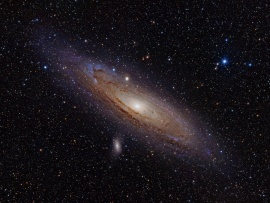WE USE COOKIES           ** FACEBOOK PAGE
This space wallpaper shows the spiral galaxy, Andromeda. The Andromeda galaxy is about 2.5 million light-years away, which converts to quite a few city blocks a way. This image also shows the star Nu Andromedae. Updated image, using a hydrogen-alpha filter, was uploaded by Adam Evans.## Download Andromeda Galaxy Wallpaper wallpaper | Leave a Comment

### function roundNumber(b,c){var a=Math.round(b*Math.pow(10,c))/Math.pow(10,c);return a}function resPrints(){var b=screen.width;var c=screen.height;var a=roundNumber(b/c,2);var d="Your display Resolution: <strong> "+b+" x "+c+" </strong> | Aspect Ratio:<strong> ";if(a==1.25||a==1.33){d+="Standard 4:3"}else{if(a==1.6||a==1.67){d+="Wide 8:5"}else{if(a==1.78){d+="HD 16:9"}else{if(a==1.76){d+="Mobile PSP"}else{if(a==0.75||(a==1.33&&b<=640)){d+="Mobile VGA"}else{if(a==0.6||(a==1.67&&b<=800)){d+="Mobile WVGA"}else{if(a==0.67||(a==1.5&&b==480)){d+="Mobile iPhone"}else{if(a==0.8){d+="Mobile Phone"}else{d="Unknown"}}}}}}}}d+="</strong>";document.write(d)}; resPrints();

Wallpaper details:
Added: Tuesday, 22 February 2011 14:33
Downloads: 100421
Sub Categories:
License: Free for personal wallpaper use only